# Unit 5 Introduction to Planning and ANN.pptx

31 May 2023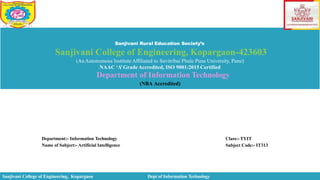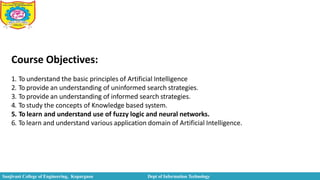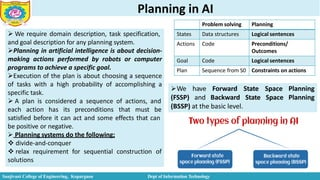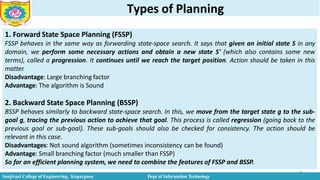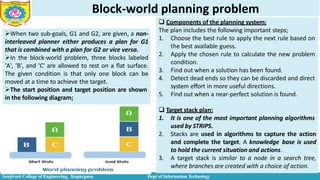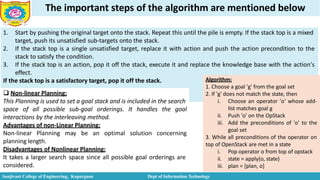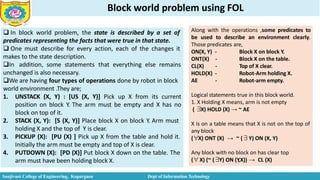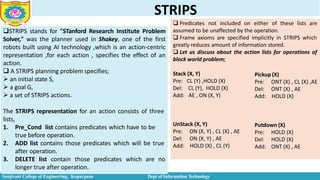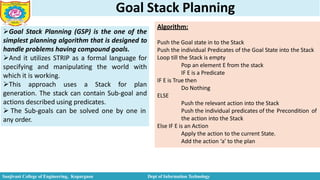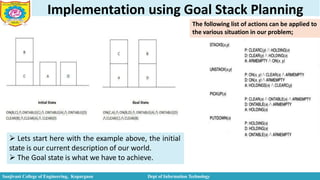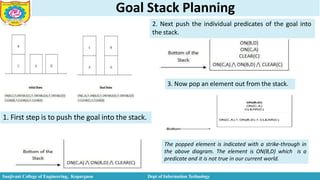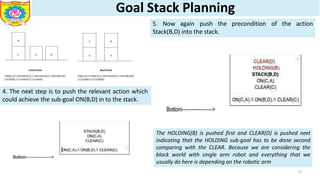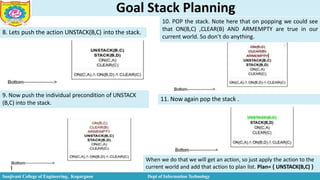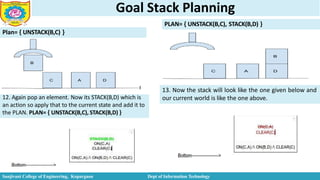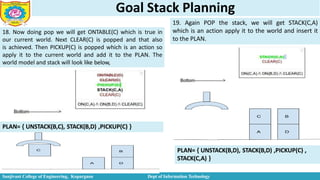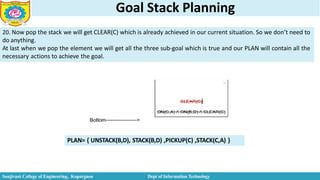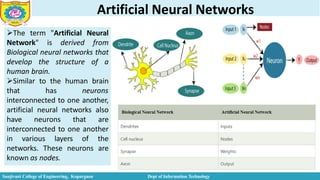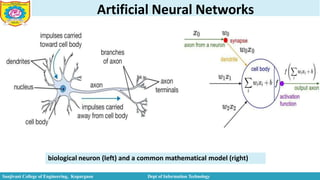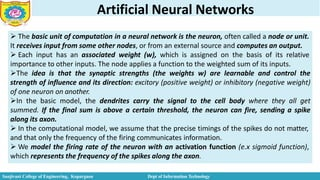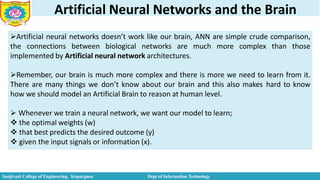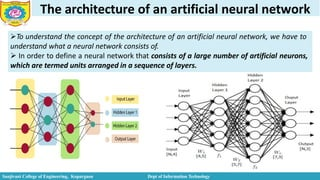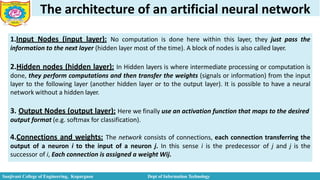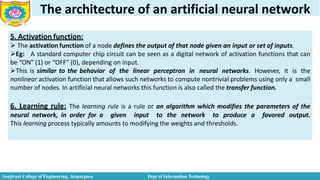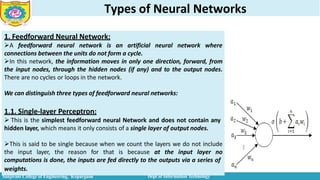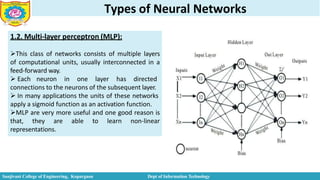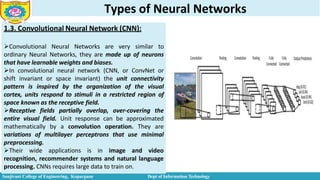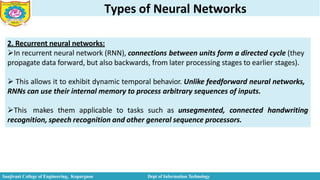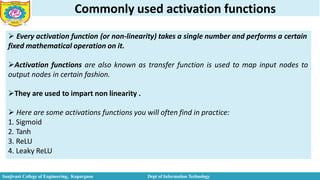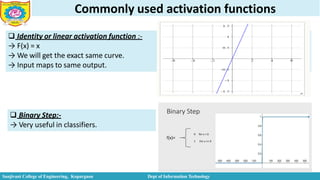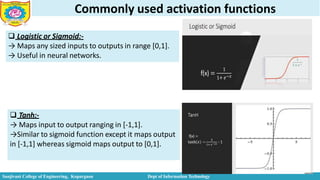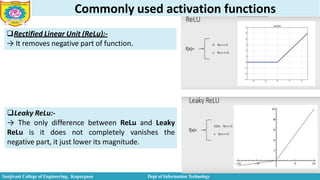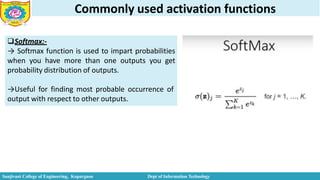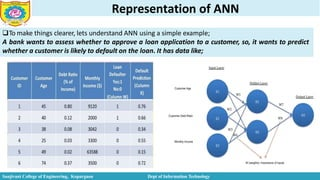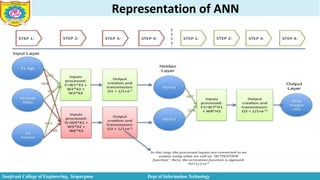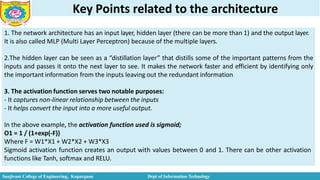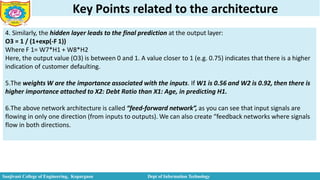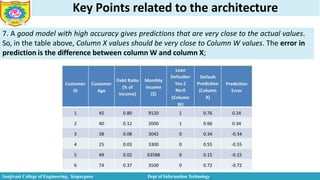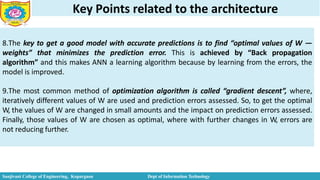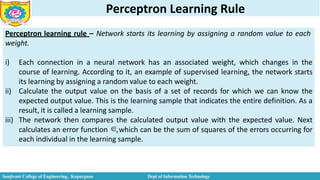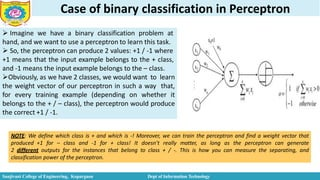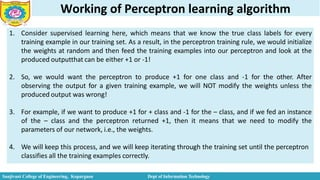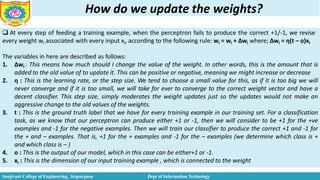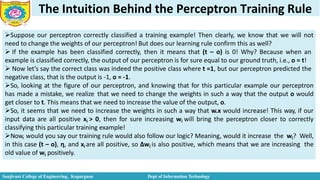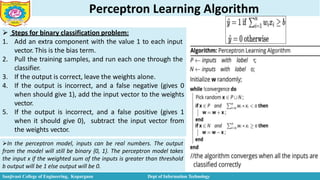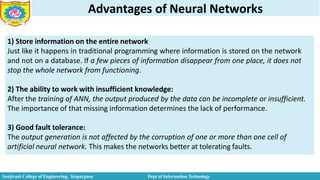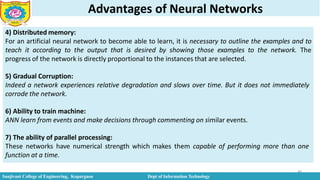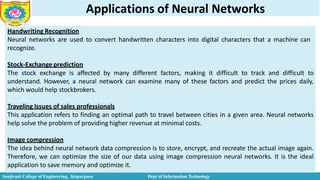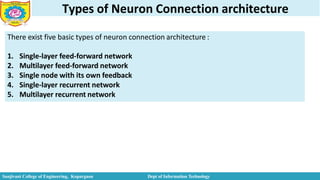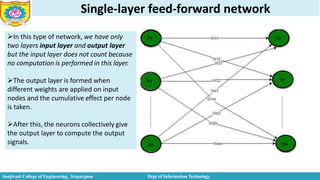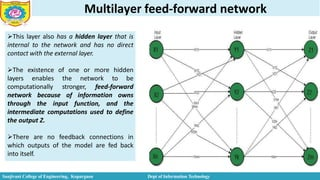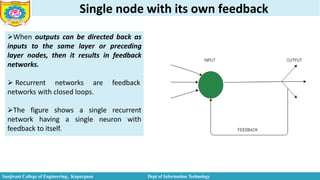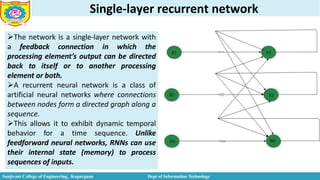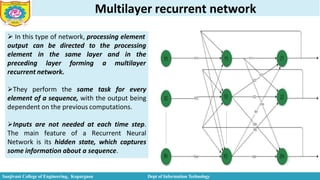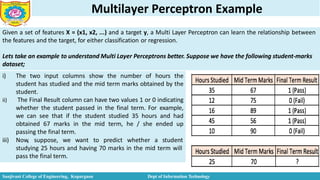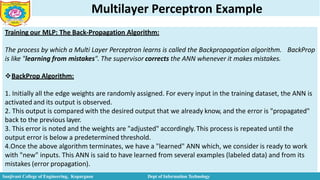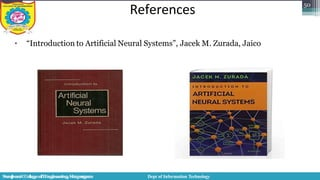1 sur 59

### Unit 5 Introduction to Planning and ANN.pptx

• 1. Sanjivani Rural Education Society’s Sanjivani College of Engineering, Kopargaon-423603 (AnAutonomous Institute Affiliated to Savitribai Phule Pune University, Pune) NAAC ‘A’GradeAccredited, ISO 9001:2015 Certified Department of Information Technology (NBA Accredited) Department:- Information Technology Name of Subject:- Artificial Intelligence Class:- TYIT Subject Code:- IT313 Sanjivani College of Engineering, Kopargaon Dept of Information Technology
• 2. Course Objectives: 1. To understand the basic principles of Artificial Intelligence 2. To provide an understanding of uninformed search strategies. 3. To provide an understanding of informed search strategies. 4. To study the concepts of Knowledge based system. 5. To learn and understand use of fuzzy logic and neural networks. 6. To learn and understand various application domain of Artificial Intelligence. Sanjivani College of Engineering, Kopargaon Dept of Information Technology
• 3. Planning in AI  We require domain description, task specification, and goal description for any planning system. Planning in artificial intelligence is about decision- making actions performed by robots or computer programs to achieve a specific goal. Execution of the plan is about choosing a sequence of tasks with a high probability of accomplishing a specific task.  A plan is considered a sequence of actions, and Sanjivani College of Engineering, Kopargaon Dept of Information Technology each action has its preconditions that must be satisfied before it can act and some effects that can be positive or negative.  Planning systems do the following;  divide-and-conquer  relax requirement for sequential construction of solutions We have Forward (FSSP) and Backward State State Space Space Planning Planning (BSSP) at the basic level. Problem solving Planning States Data structures Logical sentences Actions Code Preconditions/ Outcomes Goal Code Logical sentences Plan Sequence from S0 Constraints on actions
• 4. Types of Planning 4 1. Forward State Space Planning (FSSP) FSSP behaves in the same way as forwarding state-space search. It says that given an initial state S in any domain, we perform some necessary actions and obtain a new state S' (which also contains some new terms), called a progression. It continues until we reach the target position. Action should be taken in this matter. Disadvantage: Large branching factor Advantage: The algorithm is Sound 2. Backward State Space Planning (BSSP) BSSP behaves similarly to backward state-space search. In this, we move from the target state g to the sub- goal g, tracing the previous action to achieve that goal. This process is called regression (going back to the previous goal or sub-goal). These sub-goals should also be checked for consistency. The action should be relevant in this case. Disadvantages: Not sound algorithm (sometimes inconsistency can be found) Advantage: Small branching factor (much smaller than FSSP) So for an efficient planning system, we need to combine the features of FSSP and BSSP. Sanjivani College of Engineering, Kopargaon Dept of Information Technology
• 5. Block-world planning problem 5 When two sub-goals, G1 and G2, are given, a non- interleaved planner either produces a plan for G1 that is combined with a plan for G2 or vice versa. In the block-world problem, three blocks labeled 'A', 'B', and 'C' are allowed to rest on a flat surface. The given condition is that only one block can be moved at a time to achieve the target. The start position and target position are shown in the following diagram;  Components of the planning system: The plan includes the following important steps; 1. Choose the best rule to apply the next rule based on the best available guess. 2. Apply the chosen rule to calculate the new problem condition. 3. Find out when a solution has been found. 4. Detect dead ends so they can be discarded and direct system effort in more useful directions. 5. Find out when a near-perfect solution is found.  Target stack plan: 1. It is one of the most important planning algorithms used by STRIPS. 2. Stacks are used in algorithms to capture the action and complete the target. A knowledge base is used to hold the current situation and actions. 3. A target stack is similar to a node in a search tree, where branches are created with a choice of action. Sanjivani College of Engineering, Kopargaon Dept of Information Technology
• 6. The important steps of the algorithm are mentioned below 1. Start by pushing the original target onto the stack. Repeat this until the pile is empty. If the stack top is a mixed target, push its unsatisfied sub-targets onto the stack. 2. If the stack top is a single unsatisfied target, replace it with action and push the action precondition to the stack to satisfy the condition. 3. If the stack top is an action, pop it off the stack, execute it and replace the knowledge base with the action's effect. If the stack top is a satisfactory target, pop it off the stack.  Non-linear Planning: This Planning is used to set a goal stack and is included in the search It handles the goal space of all possible sub-goal orderings. interactions by the interleaving method. Advantages of non-Linear Planning: Non-linear Planning may be an optimal solution concerning planning length. Disadvantages of Nonlinear Planning: It takes a larger search space since all possible goal orderings are considered. Algorithm: 1. Choose a goal 'g' from the goal set Sanjivani College of Engineering, Kopargaon Dept of Information Technology 2. If 'g' does not match the state, then i. Choose an operator 'o' whose add- list matches goal g ii. Push 'o' on the OpStack iii. Add the preconditions of 'o' to the goal set 3. While all preconditions of the operator on top of OpenStack are met in a state i. Pop operator o from top of opstack ii. state = apply(o, state) iii. plan = [plan, o]
• 7. Block world problem using FOL  In block world problem, the state is described by a set of predicates representing the facts that were true in that state.  One must describe for every action, each of the changes it makes to the state description. In addition, some statements that everything else remains unchanged is also necessary. We are having four types of operations done by robot in block world environment .They are; 1. UNSTACK (X, Y) : [US (X, Y)] Pick up X from its current position on block Y. The arm must be empty and X has no block on top of it. 2. STACK (X, Y): [S (X, Y)] Place block X on block Y. Arm must holding X and the top of Y is clear. 3. PICKUP (X): [PU (X) ] Pick up X from the table and hold it. Initially the arm must be empty and top of X is clear. 4. PUTDOWN (X): [PD (X)] Put block X down on the table. The arm must have been holding block X. Along with the operations ,some predicates to be used to describe an environment clearly. Those predicates are, Sanjivani College of Engineering, Kopargaon Dept of Information Technology ON(X, Y) - ONT(X) - CL(X) - HOLD(X) - AE - Block X on block Y. Block X on the table. Top of X clear. Robot-Arm holding X. Robot-arm empty. Logical statements true in this block world. 1. X Holding X means, arm is not empty ( ∃X) HOLD (X) → ~ AE X is on a table means that X is not on the top of any block (∀X) ONT (X) → ~ (∃Y) ON (X, Y) Any block with no block on has clear top (∀X) (~ (∃Y) ON (Y ,X)) → CL (X)
• 8. STRIPS STRIPS stands for "STanford Research Institute Problem Solver," was the planner used in Shakey, one of the first robots built using AI technology ,which is an action-centric representation ,for each action , specifies the effect of an action.  A STRIPS planning problem specifies;  an initial state S,  a goal G,  a set of STRIPS actions. The STRIPS representation for an action consists of three lists, 1. Pre_Cond list contains predicates which have to be true before operation. 2. ADD list contains those predicates which will be true after operation. 3. DELETE list contain those predicates which are no longer true after operation.  Predicates not included on either of these lists are Sanjivani College of Engineering, Kopargaon Dept of Information Technology assumed to be unaffected by the operation.  Frame axioms are specified implicitly in STRIPS which greatly reduces amount of information stored.  Let us discuss about the action lists for operations of block world problem; Stack (X, Y) Pre: Del: Add: CL (Y) ,HOLD (X) CL (Y), HOLD (X) AE , ON (X, Y) UnStack (X, Y) Pre: Del: Add: ON (X, Y) , CL (X) , AE ON (X, Y) , AE HOLD (X) , CL (Y) Pickup (X) Pre: Del: Add: ONT (X) , CL (X) ,AE ONT (X) , AE HOLD (X) Putdown (X) Pre: Del: Add: HOLD (X) HOLD (X) ONT (X) , AE
• 9. Goal Stack Planning Goal Stack Planning (GSP) is the one of the simplest planning algorithm that is designed to handle problems having compound goals. And it utilizes STRIP as a formal language for specifying and manipulating the world with which it is working. This approach uses a Stack for plan generation. The stack can contain Sub-goal and actions described using predicates.  The Sub-goals can be solved one by one in any order. Algorithm: Sanjivani College of Engineering, Kopargaon Dept of Information Technology Push the Goal state in to the Stack Push the individual Predicates of the Goal State into the Stack Loop till the Stack is empty Pop an element E from the stack IF E is a Predicate IF E is True then Do Nothing ELSE Push the relevant action into the Stack Push the individual predicates of the Precondition of the action into the Stack Else IF E is an Action Apply the action to the current State. Add the action ‘a’ to the plan
• 10. Implementation using Goal Stack Planning  Lets start here with the example above, the initial state is our current description of our world.  The Goal state is what we have to achieve. The following list of actions can be applied to the various situation in our problem; Sanjivani College of Engineering, Kopargaon Dept of Information Technology
• 11. Goal Stack Planning 1. First step is to push the goal into the stack. The popped element is indicated with a strike-through in the above diagram. The element is ON(B,D) which is a predicate and it is not true in our current world. Sanjivani College of Engineering, Kopargaon Dept of Information Technology 2. Next push the individual predicates of the goal into the stack. 3. Now pop an element out from the stack.
• 12. Goal Stack Planning 4. The next step is to push the relevant action which could achieve the sub-goal ON(B,D) in to the stack. 5. Now again push the precondition of the action Stack(B,D) into the stack. The HOLDING(B) is pushed first and CLEAR(D) is pushed next indicating that the HOLDING sub-goal has to be done second comparing with the CLEAR. Because we are considering the block world with single arm robot and everything that we usually do here is depending on the robotic arm 12 K. S. Ubale Sanjivani College of Engineering, Kopargaon Dept of Information Technology
• 13. Goal Stack Planning iii) i) The popped element is HOLDING(B) which is a predicate and note that it is not true in our current world. ii) So we have to push the relevant action into the stack. In order to make the HOLDING(D) to be true there are possibly two action that can achieve it. One is PICKUP(D) and the other is UNSTACK(D,y). But now in- order to choose the best among the two actions available we have to think ahead and utilize the heuristics possibly. iv) For instance if we choose PICKUP(B) then first of all BLOCK D should be available on the table. For that we have to UNSTACK(B,D) and it will achieve HOLDING(B) which is what we want but if we use PICKUP PUTDOWN(B) making HOLDING(B) then we need to false and then use PICKUP(B) action to achieve HOLDING(B) again which can be easily achieved by using UNSTACK. v) So the best action is UNSTACK(B,y) and it also makes the current situation more close to the goal state. The variable y indicates any block below D. After popping we see that CLEAR(D) is true in the current world model so we don’t have to do anything. 7. So again pop the stack. 6. POP an element out from the stack. Sanjivani College of Engineering, Kopargaon Dept of Information Technology
• 14. Goal Stack Planning 9. Now push the individual precondition of UNSTACK (B,C) into the stack. 8. Lets push the action UNSTACK(B,C) into the stack. 10. POP the stack. Note here that on popping we could see that ON(B,C) ,CLEAR(B) AND ARMEMPTY are true in our current world. So don’t do anything. 11. Now again pop the stack . Sanjivani College of Engineering, Kopargaon Dept of Information Technology When we do that we will get an action, so just apply the action to the current world and add that action to plan list. Plan= { UNSTACK(B,C) }
• 15. Goal Stack Planning 12. Again pop an element. Now its STACK(B,D) which is an action so apply that to the current state and add it to the PLAN. PLAN= { UNSTACK(B,C), STACK(B,D) } Plan= { UNSTACK(B,C) } 13. Now the stack will look like the one given below and our current world is like the one above. PLAN= { UNSTACK(B,C), STACK(B,D) } Sanjivani College of Engineering, Kopargaon Dept of Information Technology
• 16. Goal Stack Planning 15. STACK(C,A) is pushed now into the stack and now push the individual preconditions of the action into the stack. 17. In order to achieve HOLDING(C) we have to push the action PICKUP(C) and its individual preconditions into the stack. 16. Now pop the stack. We will get CLEAR(A) and it is true in our current world so do nothing. Next element that is popped is HOLDING(C) which is not true so push the relevant action into the stack. 14. Again pop the stack. The popped element is a predicate and it is not true in our current world so push the relevant action into the stack. Sanjivani College of Engineering, Kopargaon Dept of Information Technology
• 17. Goal Stack Planning PLAN= { UNSTACK(B,C), STACK(B,D) ,PICKUP(C) } 18. Now doing pop we will get ONTABLE(C) which is true in our current world. Next CLEAR(C) is popped and that also is achieved. Then PICKUP(C) is popped which is an action so apply it to the current world and add it to the PLAN. The world model and stack will look like below, 19. Again POP the stack, we will get STACK(C,A) which is an action apply it to the world and insert it to the PLAN. Sanjivani College of Engineering, Kopargaon Dept of Information Technology PLAN= { UNSTACK(B,D), STACK(B,D) ,PICKUP(C) , STACK(C,A) }
• 18. Goal Stack Planning 20. Now pop the stack we will get CLEAR(C) which is already achieved in our current situation. So we don’t need to do anything. At last when we pop the element we will get all the three sub-goal which is true and our PLAN will contain all the necessary actions to achieve the goal. PLAN= { UNSTACK(B,D), STACK(B,D) ,PICKUP(C) ,STACK(C,A) } Sanjivani College of Engineering, Kopargaon Dept of Information Technology
• 19. Artificial Neural Networks The term "Artificial Neural Network" is derived from Biological neural networks that develop the structure of a human brain. Similar to the human brain that has neurons interconnected to one another, artificial neural networks also have neurons that are interconnected to one another in various layers of the networks. These neurons are known as nodes. Sanjivani College of Engineering, Kopargaon Dept of Information Technology
• 20. Artificial Neural Networks biological neuron (left) and a common mathematical model (right) Sanjivani College of Engineering, Kopargaon Dept of Information Technology
• 21. Artificial Neural Networks  The basic unit of computation in a neural network is the neuron, often called a node or unit. It receives input from some other nodes, or from an external source and computes an output.  Each input has an associated weight (w), which is assigned on the basis of its relative importance to other inputs. The node applies a function to the weighted sum of its inputs. The idea is that the synaptic strengths (the weights w) are learnable and control the strength of influence and its direction: excitory (positive weight) or inhibitory (negative weight) of one neuron on another. In the basic model, the dendrites carry the signal to the cell body where they all get summed. If the final sum is above a certain threshold, the neuron can fire, sending a spike along its axon.  In the computational model, we assume that the precise timings of the spikes do not matter, and that only the frequency of the firing communicates information.  We model the firing rate of the neuron with an activation function (e.x sigmoid function), which represents the frequency of the spikes along the axon. Sanjivani College of Engineering, Kopargaon Dept of Information Technology
• 22. Artificial Neural Networks and the Brain Artificial neural networks doesn’t work like our brain, ANN are simple crude comparison, the connections between biological networks are much more complex than those implemented by Artificial neural network architectures. Remember, our brain is much more complex and there is more we need to learn from it. There are many things we don’t know about our brain and this also makes hard to know how we should model an Artificial Brain to reason at human level.  Whenever we train a neural network, we want our model to learn;  the optimal weights (w)  that best predicts the desired outcome (y)  given the input signals or information (x). Sanjivani College of Engineering, Kopargaon Dept of Information Technology
• 23. The architecture of an artificial neural network To understand the concept of the architecture of an artificial neural network, we have to understand what a neural network consists of.  In order to define a neural network that consists of a large number of artificial neurons, which are termed units arranged in a sequence of layers. Sanjivani College of Engineering, Kopargaon Dept of Information Technology
• 24. The architecture of an artificial neural network 1.Input Nodes (input layer): No computation is done here within this layer, they just pass the information to the next layer (hidden layer most of the time). A block of nodes is also called layer. 2.Hidden nodes (hidden layer): In Hidden layers is where intermediate processing or computation is done, they perform computations and then transfer the weights (signals or information) from the input layer to the following layer (another hidden layer or to the output layer). It is possible to have a neural network without a hidden layer. 3. Output Nodes (output layer): Here we finally use an activation function that maps to the desired output format (e.g. softmax for classification). 4.Connections and weights: The network consists of connections, each connection transferring the output of a neuron i to the input of a neuron j. In this sense i is the predecessor of j and j is the successor of i, Each connection is assigned a weight Wij. Sanjivani College of Engineering, Kopargaon Dept of Information Technology
• 25. The architecture of an artificial neural network 5. Activation function:  The activation function of a node defines the output of that node given an input or set of inputs. Eg: A standard computer chip circuit can be seen as a digital network of activation functions that can be “ON” (1) or “OFF” (0), depending on input. This is similar to the behavior of the linear perceptron in neural networks. However, it is the nonlinear activation function that allows such networks to compute nontrivial problems using only a small number of nodes. In artificial neural networks this function is also called the transfer function. 6. Learning rule: The learning rule is a rule or an algorithm which modifies the parameters of the neural network, in order for a given input to the network to produce a favored output. This learning process typically amounts to modifying the weights and thresholds. Sanjivani College of Engineering, Kopargaon Dept of Information Technology
• 26. Types of Neural Networks 1. Feedforward Neural Network: A feedforward neural network is an artificial neural network where connections between the units do not form a cycle. In this network, the information moves in only one direction, forward, from the input nodes, through the hidden nodes (if any) and to the output nodes. There are no cycles or loops in the network. We can distinguish three types of feedforward neural networks: 1.1. Single-layer Perceptron:  This is the simplest feedforward neural Network and does not contain any hidden layer, which means it only consists of a single layer of output nodes. This is said to be single because when we count the layers we do not include the input layer, the reason for that is because at the input layer no computations is done, the inputs are fed directly to the outputs via a series of weights. Sanjivani College of Engineering, Kopargaon Dept of Information Technology
• 27. Types of Neural Networks 1.2. Multi-layer perceptron (MLP): This class of networks consists of multiple layers of computational units, usually interconnected in a feed-forward way.  Each neuron in one layer has directed connections to the neurons of the subsequent layer.  In many applications the units of these networks apply a sigmoid function as an activation function. MLP are very more useful and one good reason is that, they are able to learn non-linear representations. Sanjivani College of Engineering, Kopargaon Dept of Information Technology
• 28. Types of Neural Networks 1.3. Convolutional Neural Network (CNN): Convolutional Neural Networks are very similar to ordinary Neural Networks, they are made up of neurons that have learnable weights and biases. In convolutional neural network (CNN, or ConvNet or shift invariant or space invariant) the unit connectivity pattern is inspired by the organization of the visual cortex, units respond to stimuli in a restricted region of space known as the receptive field. Receptive fields partially overlap, over-covering the entire visual field. Unit response can be approximated mathematically by a convolution operation. They are variations of multilayer perceptrons that use minimal preprocessing. Their wide applications is in image and video recognition, recommender systems and natural language processing. CNNs requires large data to train on. Sanjivani College of Engineering, Kopargaon Dept of Information Technology
• 29. Types of Neural Networks 2. Recurrent neural networks: In recurrent neural network (RNN), connections between units form a directed cycle (they propagate data forward, but also backwards, from later processing stages to earlier stages).  This allows it to exhibit dynamic temporal behavior. Unlike feedforward neural networks, RNNs can use their internal memory to process arbitrary sequences of inputs. This makes them applicable to tasks such as unsegmented, connected handwriting recognition, speech recognition and other general sequence processors. Sanjivani College of Engineering, Kopargaon Dept of Information Technology
• 30. Commonly used activation functions  Every activation function (or non-linearity) takes a single number and performs a certain fixed mathematical operation on it. Activation functions are also known as transfer function is used to map input nodes to output nodes in certain fashion. They are used to impart non linearity .  Here are some activations functions you will often find in practice: 1. Sigmoid 2. Tanh 3. ReLU 4. Leaky ReLU Sanjivani College of Engineering, Kopargaon Dept of Information Technology
• 31. Commonly used activation functions  Identity or linear activation function :- → F(x) = x → We will get the exact same curve. → Input maps to same output.  Binary Step:- → Very useful in classifiers. Sanjivani College of Engineering, Kopargaon Dept of Information Technology
• 32. Commonly used activation functions  Logistic or Sigmoid:- → Maps any sized inputs to outputs in range [0,1]. → Useful in neural networks.  Tanh:- → Maps input to output ranging in [-1,1]. →Similar to sigmoid function except it maps output in [-1,1] whereas sigmoid maps output to [0,1]. Sanjivani College of Engineering, Kopargaon Dept of Information Technology
• 33. Commonly used activation functions Rectified Linear Unit (ReLu):- → It removes negative part of function. Leaky ReLu:- → The only difference between ReLu and Leaky ReLu is it does not completely vanishes the negative part, it just lower its magnitude. Sanjivani College of Engineering, Kopargaon Dept of Information Technology
• 34. Commonly used activation functions Softmax:- → Softmax function is used to impart probabilities when you have more than one outputs you get probability distribution of outputs. →Useful for finding most probable occurrence of output with respect to other outputs. Sanjivani College of Engineering, Kopargaon Dept of Information Technology
• 35. Representation of ANN To make things clearer, lets understand ANN using a simple example; A bank wants to assess whether to approve a loan application to a customer, so, it wants to predict whether a customer is likely to default on the loan. It has data like; Sanjivani College of Engineering, Kopargaon Dept of Information Technology
• 36. Representation of ANN Sanjivani College of Engineering, Kopargaon Dept of Information Technology
• 37. Key Points related to the architecture 1. The network architecture has an input layer, hidden layer (there can be more than 1) and the output layer. It is also called MLP (Multi Layer Perceptron) because of the multiple layers. 2.The hidden layer can be seen as a “distillation layer” that distills some of the important patterns from the inputs and passes it onto the next layer to see. It makes the network faster and efficient by identifying only the important information from the inputs leaving out the redundant information 3. The activation function serves two notable purposes: - It captures non-linear relationship between the inputs - It helps convert the input into a more useful output. In the above example, the activation function used is sigmoid; O1 = 1 / (1+exp(-F)) Where F = W1*X1 + W2*X2 + W3*X3 Sigmoid activation function creates an output with values between 0 and 1. There can be other activation functions like Tanh, softmax and RELU. Sanjivani College of Engineering, Kopargaon Dept of Information Technology
• 38. Key Points related to the architecture 4. Similarly, the hidden layer leads to the final prediction at the output layer: O3 = 1 / (1+exp(-F 1)) Where F 1= W7*H1 + W8*H2 Here, the output value (O3) is between 0 and 1. A value closer to 1 (e.g. 0.75) indicates that there is a higher indication of customer defaulting. 5.The weights W are the importance associated with the inputs. If W1 is 0.56 and W2 is 0.92, then there is higher importance attached to X2: Debt Ratio than X1: Age, in predicting H1. 6.The above network architecture is called “feed-forward network”, as you can see that input signals are flowing in only one direction (from inputs to outputs). We can also create “feedback networks where signals flow in both directions. Sanjivani College of Engineering, Kopargaon Dept of Information Technology
• 39. Key Points related to the architecture 7. A good model with high accuracy gives predictions that are very close to the actual values. So, in the table above, Column X values should be very close to Column W values. The error in prediction is the difference between column W and column X; Sanjivani College of Engineering, Kopargaon Dept of Information Technology
• 40. Key Points related to the architecture 8.The key to get a good model with accurate predictions is to find “optimal values of W — weights” that minimizes the prediction error. This is achieved by “Back propagation algorithm” and this makes ANN a learning algorithm because by learning from the errors, the model is improved. 9.The most common method of optimization algorithm is called “gradient descent”, where, iteratively different values of W are used and prediction errors assessed. So, to get the optimal W, the values of W are changed in small amounts and the impact on prediction errors assessed. Finally, those values of W are chosen as optimal, where with further changes in W, errors are not reducing further. Sanjivani College of Engineering, Kopargaon Dept of Information Technology
• 41. Perceptron Learning Rule Perceptron learning rule – Network starts its learning by assigning a random value to each weight. Sanjivani College of Engineering, Kopargaon Dept of Information Technology iii) i) Each connection in a neural network has an associated weight, which changes in the course of learning. According to it, an example of supervised learning, the network starts its learning by assigning a random value to each weight. ii) Calculate the output value on the basis of a set of records for which we can know the expected output value. This is the learning sample that indicates the entire definition. As a result, it is called a learning sample. The network then compares the calculated output value with the expected value. Next calculates an error function ∈,which can be the sum of squares of the errors occurring for each individual in the learning sample.
• 42. Case of binary classification in Perceptron  Imagine we have a binary classification problem at hand, and we want to use a perceptron to learn this task.  So, the perceptron can produce 2 values: +1 / -1 where +1 means that the input example belongs to the + class, and -1 means the input example belongs to the – class. Obviously, as we have 2 classes, we would want to learn the weight vector of our perceptron in such a way that, for every training example (depending on whether it belongs to the + / – class), the perceptron would produce the correct +1 / -1. NOTE: We define which class is + and which is -! Moreover, we can train the perceptron and find a weight vector that produced +1 for – class and -1 for + class! It doesn’t really matter, as long as the perceptron can generate 2 different outputs for the instances that belong to class + / -. This is how you can measure the separating, and classification power of the perceptron. Sanjivani College of Engineering, Kopargaon Dept of Information Technology
• 43. Working of Perceptron learning algorithm 1. Consider supervised learning here, which means that we know the true class labels for every training example in our training set. As a result, in the perceptron training rule, we would initialize the weights at random and then feed the training examples into our perceptron and look at the produced outputthat can be either +1 or -1! 2. So, we would want the perceptron to produce +1 for one class and -1 for the other. After observing the output for a given training example, we will NOT modify the weights unless the produced output was wrong! 3. For example, if we want to produce +1 for + class and -1 for the – class, and if we fed an instance of the – class and the perceptron returned +1, then it means that we need to modify the parameters of our network, i.e., the weights. 4. We will keep this process, and we will keep iterating through the training set until the perceptron classifies all the training examples correctly. Sanjivani College of Engineering, Kopargaon Dept of Information Technology
• 44. How do we update the weights?  At every step of feeding a training example, when the perceptron fails to produce the correct +1/-1, we revise every weight wi associated with every input xi, according to the following rule: wi = wi + Δwi where; Δwi = η(t – o)xi The variables in here are described as follows: 1. Δwi : This means how much should I change the value of the weight. In other words, this is the amount that is added to the old value of to update it. This can be positive or negative, meaning we might increase or decrease 2. η : This is the learning rate, or the step size. We tend to choose a small value for this, as if it is too big we will never converge and if it is too small, we will take for ever to converge to the correct weight vector and have a decent classifier. This step size, simply moderates the weight updates just so the updates would not make an aggressive change to the old values of the weights. 3. t : This is the ground truth label that we have for every training example in our training set. For a classification task, as we know that our perceptron can produce either +1 or -1, then we will consider to be +1 for the +ve examples and -1 for the negative examples. Then we will train our classifier to produce the correct +1 and -1 for the + and – examples. That is, +1 for the + examples and -1 for the – examples (we determine which class is + and which class is – ) 4. o : This is the output of our model, which in this case can be either+1 or -1. 5. xi : This is the dimension of our input training example , which is connected to the weight Sanjivani College of Engineering, Kopargaon Dept of Information Technology
• 45. The Intuition Behind the Perceptron Training Rule Suppose our perceptron correctly classified a training example! Then clearly, we know that we will not need to change the weights of our perceptron! But does our learning rule confirm this as well?  If the example has been classified correctly, then it means that (t – o) is 0! Why? Because when an example is classified correctly, the output of our perceptron is for sure equal to our ground truth, i.e., o = t!  Now let’s say the correct class was indeed the positive class where t =1, but our perceptron predicted the negative class, that is the output is -1, o = -1. So, looking at the figure of our perceptron, and knowing that for this particular example our perceptron has made a mistake, we realize that we need to change the weights in such a way that the output o would get closer to t. This means that we need to increase the value of the output, o. So, it seems that we need to increase the weights in such a way that w.x would increase! This way, if our input data are all positive xi > 0, then for sure increasing wi will bring the perceptron closer to correctly classifying this particular training example! Now, would you say our training rule would also follow our logic? Meaning, would it increase the wi? Well, in this case (t – o), η, and xi are all positive, so Δwi is also positive, which means that we are increasing the old value of wi positively. Sanjivani College of Engineering, Kopargaon Dept of Information Technology
• 46. Perceptron Learning Algorithm  Steps for binary classification problem: 1. Add an extra component with the value 1 to each input vector. This is the bias term. 2. Pull the training samples, and run each one through the classifier. 3. If the output is correct, leave the weights alone. 4. If the output is incorrect, and a false negative (gives 0 when should give 1), add the input vector to the weights vector. 5. If the output is incorrect, and a false positive (gives 1 when it should give 0), subtract the input vector from the weights vector. In the perceptron model, inputs can be real numbers. The output from the model will still be binary {0, 1}. The perceptron model takes the input x if the weighted sum of the inputs is greater than threshold b output will be 1 else output will be 0. Sanjivani College of Engineering, Kopargaon Dept of Information Technology
• 47. Advantages of Neural Networks 1) Store information on the entire network Just like it happens in traditional programming where information is stored on the network and not on a database. If a few pieces of information disappear from one place, it does not stop the whole network from functioning. 2) The ability to work with insufficient knowledge: After the training of ANN, the output produced by the data can be incomplete or insufficient. The importance of that missing information determines the lack of performance. 3) Good fault tolerance: The output generation is not affected by the corruption of one or more than one cell of artificial neural network. This makes the networks better at tolerating faults. Sanjivani College of Engineering, Kopargaon Dept of Information Technology
• 48. Advantages of Neural Networks 48 4) Distributed memory: For an artificial neural network to become able to learn, it is necessary to outline the examples and to teach it according to the output that is desired by showing those examples to the network. The progress of the network is directly proportional to the instances that are selected. 5) Gradual Corruption: Indeed a network experiences relative degradation and slows over time. But it does not immediately corrode the network. 6) Ability to train machine: ANN learn from events and make decisions through commenting on similar events. 7) The ability of parallel processing: These networks have numerical strength which makes them capable of performing more than one function at a time. Sanjivani College of Engineering, Kopargaon Dept of Information Technology
• 49. Applications of Neural Networks 4 9 Handwriting Recognition Neural networks are used to convert handwritten characters into digital characters that a machine can recognize. Stock-Exchange prediction The stock exchange is affected by many different factors, making it difficult to track and difficult to understand. However, a neural network can examine many of these factors and predict the prices daily, which would help stockbrokers. Traveling Issues of sales professionals This application refers to finding an optimal path to travel between cities in a given area. Neural networks help solve the problem of providing higher revenue at minimal costs. Image compression The idea behind neural network data compression is to store, encrypt, and recreate the actual image again. Therefore, we can optimize the size of our data using image compression neural networks. It is the ideal application to save memory and optimize it. Sanjivani College of Engineering, Kopargaon Dept of Information Technology
• 50. Types of Neuron Connection architecture There exist five basic types of neuron connection architecture : 1. Single-layer feed-forward network 2. Multilayer feed-forward network 3. Single node with its own feedback 4. Single-layer recurrent network 5. Multilayer recurrent network Sanjivani College of Engineering, Kopargaon Dept of Information Technology
• 51. Single-layer feed-forward network In this type of network, we have only two layers input layer and output layer but the input layer does not count because no computation is performed in this layer. The output layer is formed when different weights are applied on input nodes and the cumulative effect per node is taken. After this, the neurons collectively give the output layer to compute the output signals. Sanjivani College of Engineering, Kopargaon Dept of Information Technology
• 52. Multilayer feed-forward network This layer also has a hidden layer that is internal to the network and has no direct contact with the external layer. The existence of one or more hidden layers enables the network to be computationally stronger, feed-forward network because of information owns through the input function, and the intermediate computations used to define the output Z. There are no feedback connections in which outputs of the model are fed back into itself. Sanjivani College of Engineering, Kopargaon Dept of Information Technology
• 53. Single node with its own feedback When outputs can be directed back as inputs to the same layer or preceding layer nodes, then it results in feedback networks.  Recurrent networks are feedback networks with closed loops. The figure shows a single recurrent network having a single neuron with feedback to itself. Sanjivani College of Engineering, Kopargaon Dept of Information Technology
• 54. Single-layer recurrent network The network is a single-layer network with a feedback connection in which the processing element’s output can be directed back to itself or to another processing element or both. A recurrent neural network is a class of artificial neural networks where connections between nodes form a directed graph along a sequence. This allows it to exhibit dynamic temporal behavior for a time sequence. Unlike feedforward neural networks, RNNs can use their internal state (memory) to process sequences of inputs. Sanjivani College of Engineering, Kopargaon Dept of Information Technology
• 55. Multilayer recurrent network  In this type of network, processing element output element can be directed to the processing in the same layer and in the preceding layer forming a multilayer recurrent network. They perform the same task for every element of a sequence, with the output being dependent on the previous computations. Inputs are not needed at each time step. The main feature of a Recurrent Neural Network is its hidden state, which captures some information about a sequence. Sanjivani College of Engineering, Kopargaon Dept of Information Technology
• 56. Multilayer Perceptron Example Given a set of features X = (x1, x2, ...) and a target y, a Multi Layer Perceptron can learn the relationship between the features and the target, for either classification or regression. Lets take an example to understand Multi Layer Perceptrons better. Suppose we have the following student-marks dataset; i) The two input columns show the number of hours the student has studied and the mid term marks obtained by the student. ii) The Final Result column can have two values 1 or 0 indicating whether the student passed in the final term. For example, we can see that if the student studied 35 hours and had obtained 67 marks in the mid term, he / she ended up passing the final term. iii) Now, suppose, we want to predict whether a student studying 25 hours and having 70 marks in the mid term will pass the final term. Sanjivani College of Engineering, Kopargaon Dept of Information Technology
• 57. Multilayer Perceptron Example Training our MLP: The Back-Propagation Algorithm: The process by which a Multi Layer Perceptron learns is called the Backpropagation algorithm. BackProp is like "learning from mistakes". The supervisor corrects the ANN whenever it makes mistakes. BackProp Algorithm: 1. Initially all the edge weights are randomly assigned. For every input in the training dataset, the ANN is activated and its output is observed. 2. This output is compared with the desired output that we already know, and the error is "propagated" back to the previous layer. 3. This error is noted and the weights are "adjusted" accordingly. This process is repeated until the output error is below a predetermined threshold. 4.Once the above algorithm terminates, we have a "learned" ANN which, we consider is ready to work with "new" inputs. This ANN is said to have learned from several examples (labeled data) and from its mistakes (error propagation). Sanjivani College of Engineering, Kopargaon Dept of Information Technology
• 58. References Dept of Information Technology S S a a n n j j i i v v a a n n i iC C o o l l l l e e g g e eo o f fE E n n g g i i n n e e e e r r i i n n g g , ,K K o o p p a a r r g g a a o o n n 50 • “Introduction to Artificial Neural Systems”, Jacek M. Zurada, Jaico
• 59. Thank you Sanjivani College of Engineering, Kopargaon Dept of Information Technology